ZeitschriftendatenFormat
Zeitschrift
eISSN
2083-831X
Erstveröffentlichung
09 Nov 2012
Erscheinungsweise
4 Hefte pro Jahr
Sprachen
EnglischUneingeschränkter Zugang

# An iterative algorithm for random upper bound kinematical analysis

###### Akzeptiert: 27 Sep 2021
ZeitschriftendatenFormat
Zeitschrift
eISSN
2083-831X
Erstveröffentlichung
09 Nov 2012
Erscheinungsweise
4 Hefte pro Jahr
Sprachen
Englisch

A new approach for stochastic upper bound kinematical analyses is described. The study proposes an iterative algorithm that uses the Vanmarcke spatial averaging and kinematical failure mechanisms. The iterative procedure ensures the consistency between failure geometry and covariance matrix, which influences the quality of the results. The proposed algorithm can be applied to bearing capacity evaluation or slope stability problems. The iterative algorithm is used in the study to analyse the three-dimensional undrained bearing capacity of shallow foundations and the bearing capacity of the foundation for two-layered soil, in both cases, the soil strength spatial variability is included. Moreover, the obtained results are compared with those provided by the algorithm, based on the constant covariance matrix. The study shows that both approaches provide similar results for a variety of foundation shapes and scale of fluctuation values. Therefore, the simplified algorithm can be used for purposes that require high computational efficiency and for practical applications. The achieved efficiency using a constant covariance matrix for one realisation of a three-dimensional bearing capacity problem that includes the soil strength spatial variability results in about 0.5 seconds for a standard notebook. The numerical example presented in the study indicates the importance of the iterative algorithm for further development of the failure mechanism application in probabilistic analyses. Moreover, because the iterative algorithm is based on the upper bound theorem, it could be utilised as a reference for other methods for spatially variable soil.

Introduction

Stochastic analyses are currently used extensively in a wide range of geotechnical applications. This is the reason of soil spatial variability caused by the geological processes that form the soil layer (e.g., Ferreira et al., 2015). The influence of soil spatial variability is examined via numerical methods based on the finite element method, finite difference method, or finite limit analysis. These methods are used to examine the impact of soil strength spatial variability on engineering structures such as foundations, slopes, or sheet pile walls (e.g., Griffiths et al., 2002; Fenton and Griffiths, 2002, 2008; Griffiths and Fenton, 2004; Srivastava et al., 2010; Kasama and Whittle, 2011; Huang et al., 2013; Simoes et al., 2014; Zhu et al, 2017; Kawa et al., 2019; Halder and Chakraborty, 2020; Pramanik et al., 2020; Żyliński et al., 2020; Kawa and Puła 2020; Viviescas et al., 2020; Pramanik et al., 2021). However, the numerical efficiency of most existing probabilistic methods is not sufficient for practical applications where three-dimensional analyses are crucial. This is true especially for three-dimensional issues for which the three-dimensional nature of soil strength spatial variability suggests that there are difficulties with its two-dimensional simplifications (e.g. plane strain conditions). This is the reason researchers investigated the possibility of providing more efficient 3-D approaches for bearing capacity analysis in the case of soil spatial variability, e.g., Chwała (2019; 2020), Li et al. (2021), Li et al., (2021). In the earlier papers by Puła and Chwała (2018) and Chwała (2019), the algorithm for using the upper bound limit theorem and Vanmarcke's spatial averaging (Vanmarcke, 1977a,b; 1983) is presented for two-dimensional and three-dimensional bearing capacity evaluation, respectively. However, the numerical efficiency achieved by Chwała (2019) can be further improved by using the so-called constant covariance matrix approach (Chwała, 2020). The constant covariance matrix approach means that the matrix is obtained using the expected values of soil strength parameters; the concept was first proposed by Puła (2004; 2007). Generally speaking, the above approaches are based on random field discretisation to a single random variable (each random variable corresponds to a specific dissipation region in a failure mechanism). These variables are correlated with the covariance matrix, and this matrix is the basis for generating the average soil strength parameters (e.g., Fenton and Griffiths, 2008; Puła and Chwała, 2015). However, the solution suggested by Chwała (2019) for three-dimensional issues is based on one iteration of the covariance matrix (for more details see the next section). The same approach is used for two-dimensional analyses by Puła and Chwała (2018). Therefore, there is still a need of verify this assumption. To study the impact of the iteration number of covariance matrix and to extend the use of the proposed method to other geotechnical applications, an iterative algorithm was developed and is described in this study. The main objective is to provide a general procedure that can be utilised for a variety of geotechnical applications, where the upper bound limit theorem (e.g., Shield and Drucker, 1953) and Vanmarcke's spatial averaging are used. Another important objective of this study is to compare the simplified approach, which is based on the constant covariance matrix with the iterative procedure that is proposed here. The iterative procedure is based on redetermining the covariance matrix in the subsequent iterations; thus, the covariance matrix corresponds to the failure geometry. As a numerical example, the proposed algorithm is used to analyse the three-dimensional bearing capacity of a shallow foundation under undrained conditions and the bearing capacity of two-layered soils. The comparison of the iterative approach with the constant matrix approach is crucial for further practical applications of the simplified algorithm for three-dimensional issues like soil sounding location optimization (Chwała, 2020; 2021). This comparison is important due to the enormous reduction in computation time. Namely, three-dimensional analyses that were performed in this study for a constant covariance matrix took about 0.5 s using a standard notebook. Therefore, there is a great potential to improve the numerical efficiency in the three-dimensional probabilistic analyses by using the constant covariance matrix approach. However, the iterative procedure is needed to examine the impact of this assumption on the resulting bearing capacity probabilistic characteristics.

Numerical algorithm

The proposed procedure is devoted to random analyses of geotechnical problems in the framework of the upper bound limit theorem. Its engineering applications are directed toward foundation bearing capacity evaluation and slope stability evaluation for spatialy variable soil. The procedure is described in as general manner as possible; however, some information directed to the examples used in the study are given. Two problems are investigated employing the proposed iterative algorithm, i.e., the algorithm proposed by Chwała (2019) for 3-D bearing capacity analysis, and the algorithm proposed by Chwała and Puła (2020) and Chwała and Kawa (2021) for two-layered soil bearing capacity calculation. As mentioned in the previous section, the procedure is based on the determination of the covariance matrix, which corresponds to the actual geometry of failure. The components of the covariance matrix are established via Vanmarcke spatial averaging, and the necessary formulae have to be derived independently for each problem (those for the three-dimensional bearing capacity are given by Chwała (2019), and for two-layered soil by Chwała and Puła (2020)). As indicated earlier, the above-mentioned algorithms use one iteration of the covariance matrix, meaning that after determination of the covariance matrix (which is based on the failure geometry that was obtained for uncorrelated soil strength parameters, see details in the algorithm description below), the correlated soil strength parameters are generated. Then, they are used to find the optimal failure geometry and the corresponding final bearing capacity. However, there is an inconsistency in the above approach, which is that the optimal failure geometry differs from the failure geometry for which the covariance matrix was established. This may introduce additional uncertainty in the observed results, which can be eliminated by the iterative procedure that is proposed in this study. In the algorithm detailed below, the covariance matrix is determined as many times as the assumed number of iterations N. The difference between the subsequent covariance matrices decreases when the number of iterations increases. To ensure the generalisability of the algorithm (i.e., the possibility that it can be used for various geotechnical problems), its layout is as universal as possible. For further discussion, the following algorithm is denoted as ‘A’.

Step 1. Preliminary assumptions. At the beginning stage, information about the initial random field characteristics of the soil spatial variability, is required. This information consists of a mean value μ, the point variance σ2, the type of probability density function, and the scale of fluctuation θ (or scales of fluctuation if they are distinguished in the vertical and horizontal directions). Moreover, the random field correlation structure is assumed; the most commonly used covariance functions are Gaussian, see Eq. (1), and Markov, see Eq. (2) (see Fenton and Griffiths, 2008). The stationary and separable random fields are assumed in the algorithm. $R(Δx,Δy,Δz)=σ2exp{ −[ (πΔxθx)2+(πΔyθy)2+(πΔzθz)2 ] }$ R\left( {\Delta x,\Delta y,\Delta z} \right) = {\sigma ^2}\exp \left\{ { - \left[ {{{\left( {{{\sqrt \pi \Delta x} \over {{\theta _x}}}} \right)}^2} + {{\left( {{{\sqrt \pi \Delta y} \over {{\theta _y}}}} \right)}^2} + {{\left( {{{\sqrt \pi \Delta z} \over {{\theta _z}}}} \right)}^2}} \right]} \right\} $R(Δx,Δy,Δz)=σ2exp{ −[ 2| Δx |θx+2| Δy |θy+2| Δz |θz ] }$ R\left( {\Delta x,\Delta y,\Delta z} \right) = {\sigma ^2}\exp \left\{ { - \left[ {{{2\left| {\Delta x} \right|} \over {{\theta _x}}} + {{2\left| {\Delta y} \right|} \over {{\theta _y}}} + {{2\left| {\Delta z} \right|} \over {{\theta _z}}}} \right]} \right\} where, θx, θy, and θz are scales of fluctuation in the specified directions, and Δx, Δy, Δz are distances along x, y and z directions. If two soil strength parameters are described by random fields (e.g. angle of internal friction and cohesion), the correlation ratio ρ between them can be included in the analyses (in this case, the size of the covariance matrix from step 4 is enlarged; for more details see Puła and Chwała, 2015). Next, the corresponding failure mechanism has to be chosen or established. The failure geometry has to be kinematically admissible according to the upper bound theorem.

Step 2. Generation of independent soil strength parameters. According to the initial random field characteristics (μ, σ2, and the type of probability density function), an appropriate number of independent soil strength parameters γ0=γi0 are generated using a random number generator. This number is equal to the number of dissipation regions in the considered failure mechanism n (i=1,…,n). If two soil strength parameters are considered, both values are generated for each dissipation region.

Step 3. Determination of the optimal failure geometry. For soil parameters γ0, the value of the corresponding upper bound limit load p0 (bearing capacity) is computed based on the total energy dissipation in the failure mechanism. However, it is necessary to find the minimum upper bound limit load, and the optimal failure geometry is searched using a dedicated optimisation procedure. Thus, the optimal failure geometry Δ0 and the corresponding upper bound limit load p0 are determined. The optimisation procedure should be chosen individually for the considered problem, and there are no specific restrictions on this issue.

Step 4. Determination of covariance matrix and generation of correlated soil strength parameters. The covariance matrix C0 is determined based on the optimal failure geometry Δ0. The size of the matrix is n × n (or 2n × 2n if two correlated soil strength parameters are considered), and the matrix is symmetrical and positive definite (thus, the Cholesky decomposition exists, see e.g., Horn, 1985). The components of the covariance matrix between two dissipation regions can be derived from the following formula (for more details see Puła 2004; 2007 or Puła and Chwała, 2015): $Cov(Xi,Xj)=1| V1 || V2 |∫V1∫V2R(xi,yi,zi,xj,yj,zj)dVi(xi,yi,zi)dVj(xj,yj,zj)$ \matrix{{{\rm{Cov}}\left( {{X_i},{X_j}} \right) = {1 \over {\left| {{V_1}} \right|\left| {{V_2}} \right|}} {\int_{{V_1}}} {\int_{{V_2}}R\left( {{x_i},{y_i},{z_i},{x_j},{y_j},{z_j}} \right)} } \cr {d{V_i}\left( {{x_i},{y_i},{z_i}} \right)d{V_j}\left( {{x_j},{y_j},{z_j}} \right)} \cr } Where indexes i and j run from 1 to the number of dissipation regions n in the failure mechanism, and V1 and V2 are the integration regions (section, surface, or volume). Based on C0, the independent soil strength parameter vector γ0 is transformed into the correlated parameter vector γC1 by calculating the product of standardized γ0 vector and triangular matrix which is the result of Cholesky decomposition of the covariance matrix C0 (for more details, see Puła and Chwała, 2015 or Chwała 2019).

Step 5. Beginning of the iterative procedure. Set k=1, and proceed to step 6.

Step 6. Set k=k+1. Using the optimisation procedure from step 3 for the correlated soil parameters γCk, the optimal failure geometry Δk and corresponding upper bound limit load pk are calculated.

Step 7. Determine the covariance matrix Ck for the optimal failure geometry Δk. Based on Ck, the independent soil strength parameter γ0 is transformed into the correlated parameter γCk+1 using the covariance matrix Ck.

Step 8. Using the optimisation procedure from step 3 for the soil parameters γCk+1, the optimal failure geometry Δk+1 and corresponding upper bound limit load pk+1 are calculated.

Step 9. If kkmax, proceed to step 10; otherwise, proceed to step 6. The maximum number of iterations kmax can be assumed arbitrarily after investigation of the convergence of the results. Additionally, different conditions for stopping the procedure are possible.

Step 10. For the end of the numerical procedure, the last values of the vector Δkmax and pkmax are the optimal failure geometry and the corresponding upper bound limit load, respectively.

First, the above steps of the algorithm can be developed and adjusted in an individual pattern to be suitable for specified issues that are examined, such as slope stability or foundation bearing capacity.

Steps 2 to 10 are repeated N times, where N denotes the number of Monte Carlo realisations. Generally, the choice of the number N determines the accuracy that is obtained, i.e., a greater N provides more accurate estimations. Thus, a reasonable compromise between accuracy and computation time must be established. The overall computational efficiency can be improved using a framework that is characterised by faster convergence, such as subset simulation (Au and Beck, 2001).

As discussed above, the difference between subsequent covariance matrices Ck and Ck+1 is expected to decrease when the iteration number k increases. This suggests that there is a decreasing tendency in the differences between subsequent bearing capacities pk and pk+1 and failure geometry parameters Δk,i and Δk+1,i. The tendency can be expressed by Eq. (4). $limk→∞pkpk+1=limk→∞Δk,iΔk+1,i=1.0$ \mathop {\lim }\limits_{k \to \infty } {{{p_k}} \over {{p_{k + 1}}}} = \mathop {\lim }\limits_{k \to \infty } {{{\Delta _{k,i}}} \over {{\Delta _{k + 1,i}}}} = 1.0 The values resulting from Eq. (4) are influenced by numerical errors that are caused by finite numerical accuracy and the procedure that ensuring the positivity of the covariance matrix. However, a specified iteration number k can be found, for which the difference between two subsequent values from Eq. (4) becomes negligible or equal to the numerical accuracy. Moreover, due to the results described in the next sections, the stabilisation is observed for a relatively small value of k. An increase in the iteration number kmax causes a proportional decrease in computational efficiency. However, for some engineering problems, the differences between p1 and pk (where k→∞) should be relatively small or even negligible. Therefore, in such cases, the computational efficiency can be significantly improved using only one or two covariance matrix iterations. One covariance matrix can also be determined for all Monte Carlo simulations, and the natural choice is to determine it for the failure geometry, which corresponds to the expected values of the soil strength parameters. The idea was first proposed by Puła (2004) and discussed later for a two-dimensional Prandtl failure mechanism by Puła and Chwała (2015). In this study, a simple general algorithm for using a constant covariance matrix is proposed and used for three-dimensional bearing capacity evaluation and bearing capacity of two-layered soil. In the numerical example section, results for both approaches are discussed. For each case, the algorithm that is based on the constant covariance matrix may provide sufficient accuracy for engineering practice, and it provides a dramatic improvement in computational efficiency. However, a previous investigation is required if the constant covariance matrix is to be used in numerical analyses. To illustrate the algorithm layout, the flowcharts are shown in Fig. 1. The path denoted by ‘A’ represents the ten steps described above, and the path ‘B’ represents a slight modification of ‘A’, where only Step 3 differs. The difference is a distinct way to determine the optimal failure geometry, i.e., in the path ‘B’ the optimal failure geometry is established for the expected values of the soil strength parameters. Path ‘B’ can be utilised to speed up the convergence of the results when the iterative procedure is used. The distinct path ‘C’ is the algorithm in which a constant covariance matrix is utilised (the covariance matrix in Step 3 in the path ‘C’ is determined only once for all Monte Carlo simulations).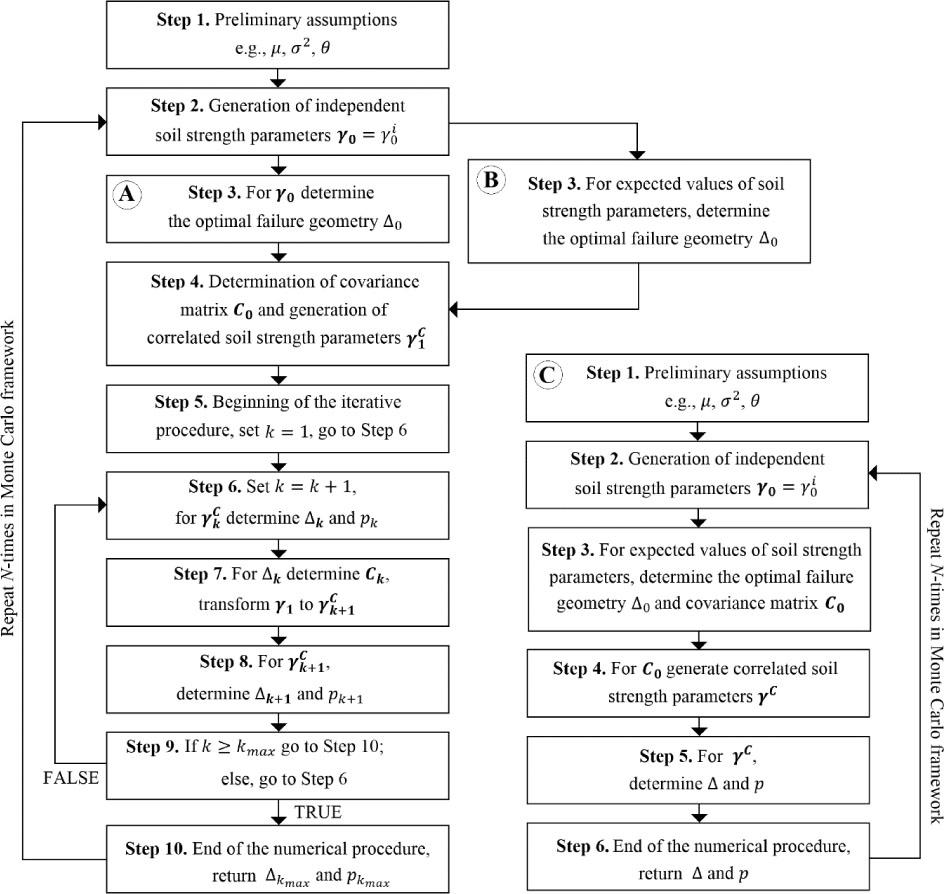The three algorithms discussed in the study. Path ‘A’ is the base iterative algorithm, which is described in detail in the text, the path ‘B’ differs only from ‘A’ in Step 3 (see the description in the text), and the path ‘C’ is dedicated to a constant covariance matrix. Both ‘A’, ‘B’, and ‘C’ are repeated N times in the Monte Carlo framework.
Application of the iterative algorithm – bearing capacity of two-layered soil
Preliminary assumptions and problem definition

As the first numerical example, the random bearing capacity of two-layered soil was analysed via the iterative algorithm described above. The optimisation procedure and formulae for the covariance matrix components were assumed according to a previous study (Chwała and Puła, 2020) and are not repeated here. Thus, the optimisation procedure that is based on the simulation annealing (Kirkpatrick et al., 1983; Kirkpatrick, 1984) was used to search for the optimal failure geometry (the geometry for which the bearing capacity reaches its minimum). The corresponding failure geometry that is adapted to the probabilistic analyses is shown in Fig. 2. Note that the failure mechanism shown in Fig. 2 is for the case of the homogenous sand layer as the top layer and spatially variable clay as a bottom layer. This scenario describes the situation of working platforms, where the top layer homogeneity results from a man-made layer. In this example, the boundary between two soil layers is assumed to be deterministic, i.e., its depth is constant in Monte Carlo analyses; however, generally, this issue is more complicated (e.g., Ghanem and Brząkała, 1996; Bagińska et al., 2020; Rainer and Szabowicz, 2020).

First, as a numerical procedure, the iterative algorithm that is denoted by ‘A’ in Fig. 1 was used. The assumed iteration number is k=6; thus, six values of the bearing capacity pk are obtained for each Monte Carlo simulation (k=1,…,6). According to a study by Chwała and Puła (2020) and Fig. 2, there are 16 dissipation regions in the failure mechanism; thus, in Step 2, 16 independent values of undrained shear strength are generated, and as a result, the size of the covariance matrix is 16 × 16. Note that the considered failure mechanism is for plane-strain conditions; however, the spatial averaging is performed in three dimensions. The Markovian covariance function is given in Eq. (2) is used in determining the covariance matrix component. The results obtained using algorithm ‘A’ were juxtaposed with those obtained using algorithm ‘C’ (see Fig. 1). As mentioned above, the algorithm ‘C’ relies on the constant covariance matrix concept, which means that the covariance matrix is determined only once for the expected soil strength parameter values (here, the parameters are the expected values of the undrained shear strength). To identify the differences between both approaches, the results for both algorithms were combined and are shown in the same figure. Therefore, for the algorithm ‘C’, k=0 is assigned; however, for algorithm ‘A’; k=1, k=2, k=3, k=4, k=5, and k=6 are assigned (depending on the iteration number).

Analysed scenarios

Eight scenarios were considered for the bearing capacity of two-layered soil. Two averaging lengths are considered (they can be interpreted as shallow foundation lengths), i.e., a a=2.0 m and a=8.0 m. The anisotropic correlation structure in the undrained shear strength random field is assumed. The following scales of fluctuation were included in the analyses, for the vertical one: θv = 0.5 m, and θv = 1 m, and for the horizontal one: θh = 2 m, and θh= 10 m. The values of vertical scale of fluctuation were motivated by the results obtained from field investigations and earlier studies (e.g., Phoon and Kulhawy, 1999; Stuedlein et al., 2012; Bagińska et al., 2016; Pieczyńska-Kozłowska et al., 2017). Note that analysing correlation length for spatially variable soil is a challenging task that still attracts researchers interest (e.g., Ching et al., 2018; Viviescas et al., 2021). The undrained shear strength was described via a lognormal random field characterised by the Markovian covariance function (Eq. 2), with the mean value μcu = 9 kPa and coefficients of variation vcu = 0.5. In this scenario, a linear trend in undrained shear strength is also considered. The included trend is 9 kPa/m starting from the bottom layer. The trend consideration is based on the recent algorithm by Chwała and Kawa (2021). For the homogenous sand layer, the friction angle ϕ=35°, and cohesion 1 kPa are assumed.

Results

According to the algorithms presented in Fig. 1, the number of realisations N must be accepted. In this study, the number N equals 200 for both two-layered soil and three-dimensional case. This relatively low value is due to the intention of comparison between both approaches, but not the precise estimation of the mean and standard deviation of bearing capacity. To make comparison possible, the same set of independent soil strength parameters (see Step 2 in Fig. 1) is used in algorithm ‘A’ and algorithm ‘C’. Eight randomly selected results among 200 Monte Carlo realizations for the case of θh=2 m, θv=1 m and a=2 m are shown in Fig. 3. The greatest differences in bearing capacity are observed between the constant covariance matrix (k=0) and the first iteration in algorithm ‘A’ (k=1) and between the first and the second iterations in algorithm ‘A’ (k=1, k=2). For the next iterations, the values of bearing capacity tend to stabilize.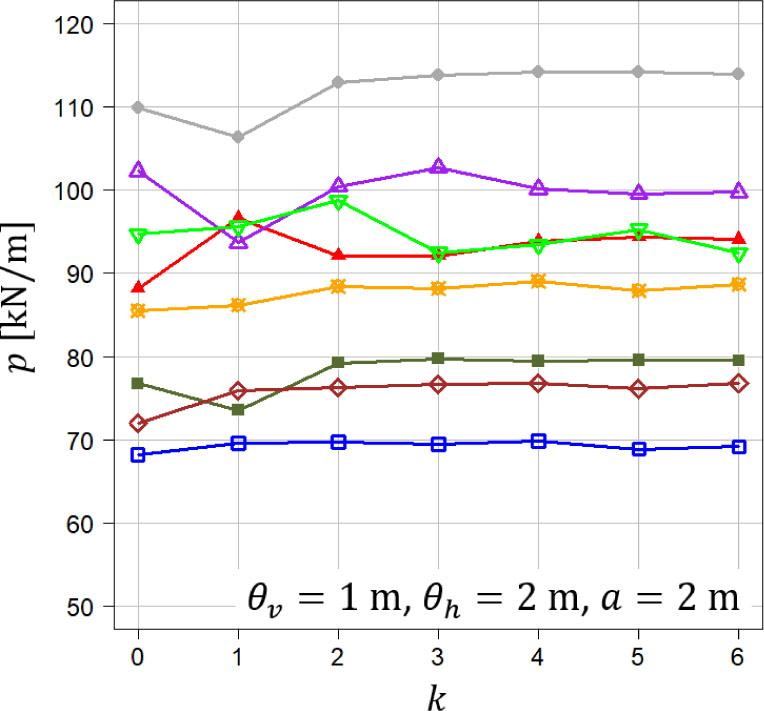Eight randomly selected results among 200 Monte Carlo realizations for the case of θh=2 m, θv=1 m, and a=2 m.

The obtained results for two-layered soil provided as bearing capacity mean values and standard deviations are shown in Fig. 4a and Fig 4b, respectively.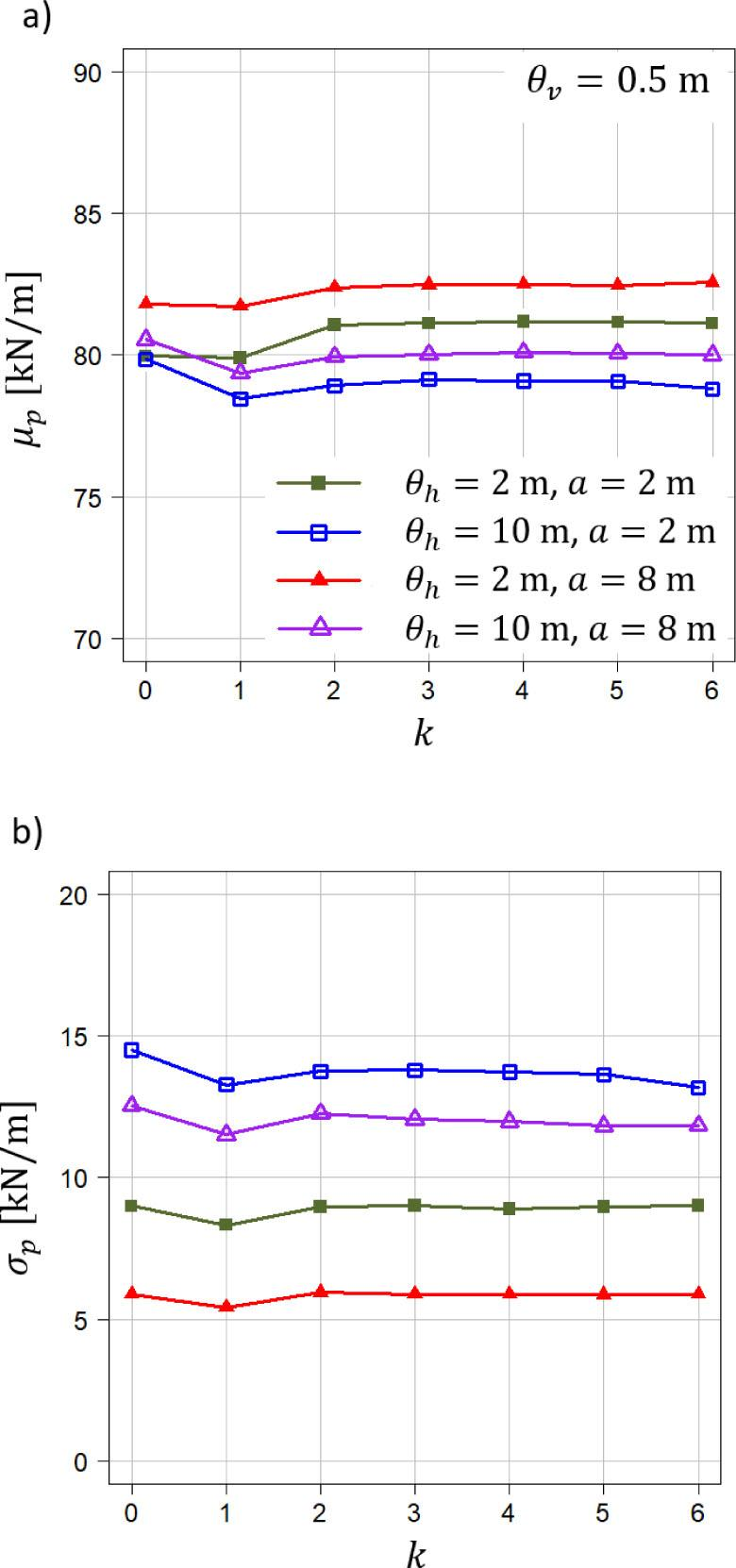Bearing capacity mean values as a function of covariance matrix iteration number (a). Bearing capacity standard deviations as a function of covariance matrix iteration number (b). Case k=0 is for the constant covariance matrix. Results for two-layered soil, vertical scale of fluctuation θv=0.5 m,, foundation width b=1 m and averaging lengths a=2 m and a=8 m.

As shown in Fig. 4a, the bearing capacity mean values are in a narrow range, the greatest differences occur in a range for k from 0 to 2 (what corresponds to the effect shown in Fig. 3 for individual realizations); however, the bearing capacity mean values are almost constant for k in a range from 2 to 6. This means that further covariance matrix iteration has no significant impact on the mean value estimates in the considered example, as shown in Fig. 4b, the analogous situation is observed in the case of bearing capacity standard deviation. Analogous reslts for for vertical scale of fluctuation θv=1 m are shown in Fig. 5. In Fig. 5a and Fig, 5b, the bearing capacity mean value, and bearing capacity standard deviations are shown, respectively.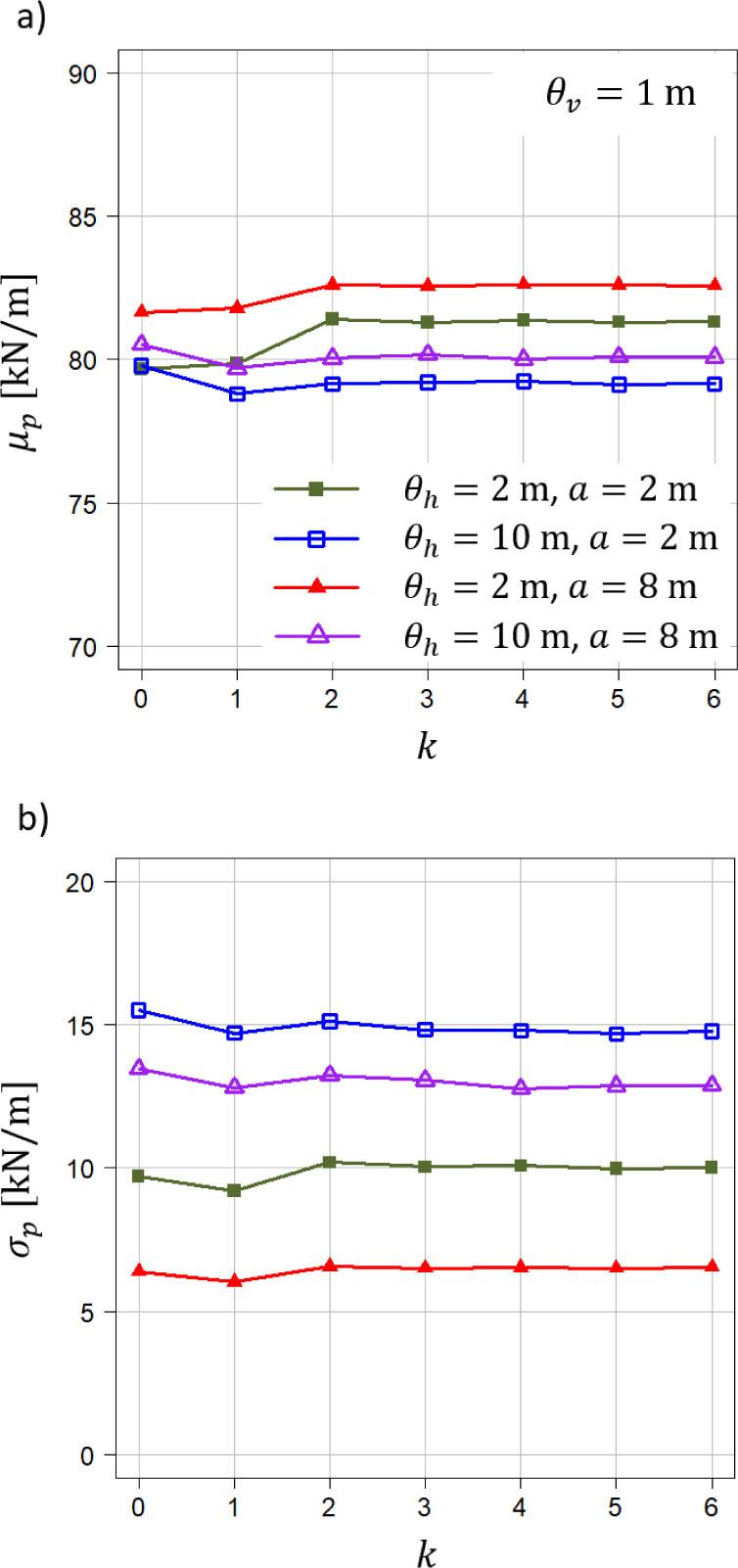Bearing capacity mean values as a function of covariance matrix iteration number (a). Bearing capacity standard deviations as a function of covariance matrix iteration number (b). Case k=0 is for the constant covariance matrix. Results for two-layered soil, vertical scale of fluctuation θv=1 m,, foundation width b=1 m and averaging lengths a=2 m and a=8 m.

As shown in Fig. 5, both the bearing capacity mean value and standard deviation are very close to each other for all considered values of k. The greatest differences in Fig. 4a and Fig. 5a are observed for bearing capacity mean values for the horizontal scale of fluctuation θh=2 m and averaging length a=2 m (case k=0 and k≥2; and case, k=1 and k≥2). The obtained standard deviation curves (see Fig. 4b and Fig. 5b) are similar for all cases.

Application of the iterative algorithm – three-dimensional bearing capacity for undrained conditions
Preliminary assumptions and problem definition

As the second numerical example, the random bearing capacities of square and rectangular foundations were analysed via the iterative algorithm described above. The optimisation procedure and formulae for the covariance matrix components were assumed according to a previous study (Chwała, 2019) and are not repeated here. Thus, the optimisation procedure that is based on the simulation annealing (Kirkpatrick et al., 1983; Kirkpatrick, 1984) was used to search for the optimal failure geometry (the geometry for which the bearing capacity reaches its minimum). A rough foundation base is assumed, and therefore, the corresponding failure geometry is adapted to the probabilistic analyses, and is shown in Fig. 6.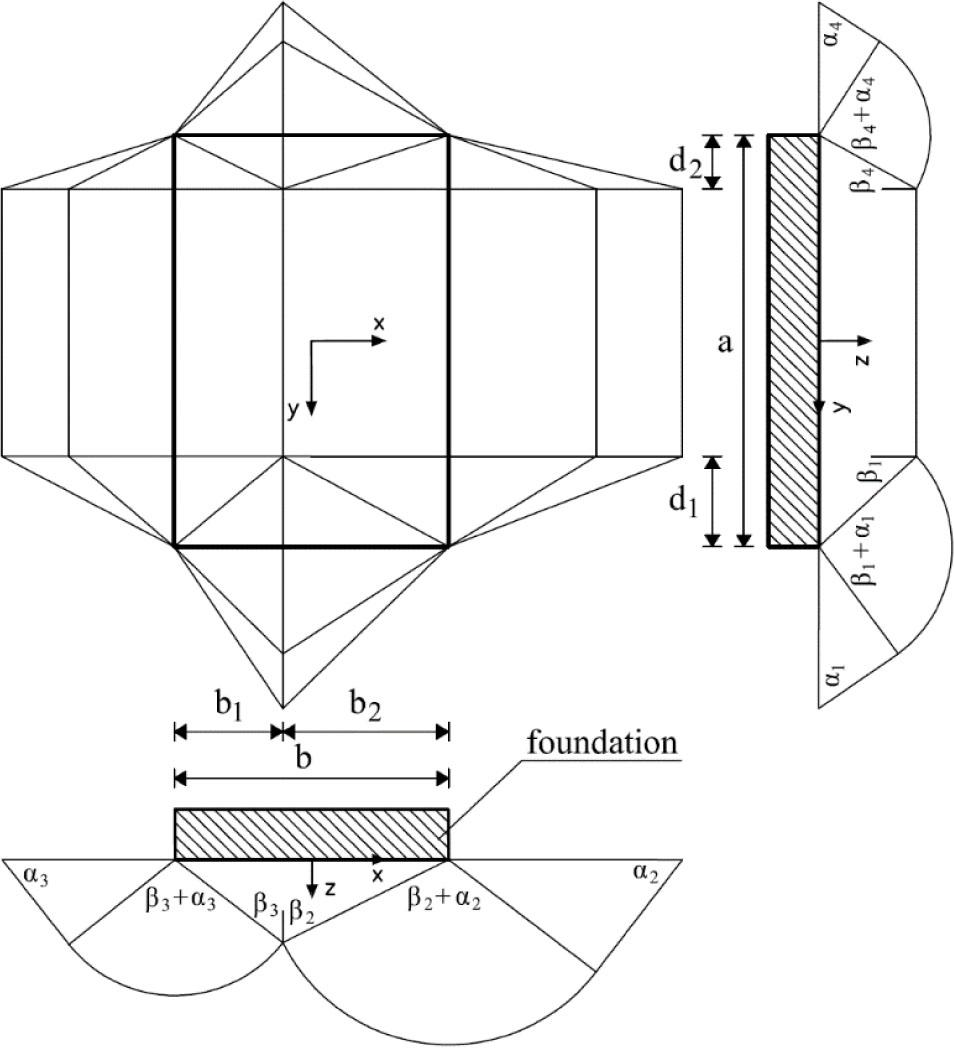Three-dimensional failure geometry of the rough foundation base for the probabilistic case. The indicated angles and lengths are used to determine the failure geometry.

First, as a numerical procedure, similarly as in the previous example, the iterative algorithm ‘A’ (see Fig. 1) is used. The assumed iteration number is k=6; thus, six values of the bearing capacity pk are obtained for each Monte Carlo realisation (k=1,…,6). According to the study by Chwała (2019) and Fig. 6, there are 30 dissipation regions in the failure mechanism; thus, in Step 2, 30 independent values of undrained shear strength are generated (the size of the covariance matrix is 30 × 30). The Gaussian covariance function given in Eq. (1) is used in determining the covariance matrix components. The results obtained by algorithm ‘A’ were juxtaposed with those obtained using algorithm ‘C’ (algorithm ‘C’ is based on a constant covariance matrix, see Fig. 1). To identify the differences between both approaches, the results for both algorithms were combined and are shown in the same figures. Therefore, for the algorithm ‘C’, k=0 is assigned; however, for algorithm ‘A’; k=1, k=2, k=3, k=4, k=5, and k=6 are assigned (depending on the iteration number).

Analysed scenarios

Six scenarios were considered for the three-dimensional failure mechanism. Two shapes of the shallow foundation are assumed, i.e., square foundation (b=1.0 m and a=1.0 m) and rectangular foundation (b=1.0 m and a=4.0 m). The isotropic correlation structure of the random field (which describes undrained shear strength) was examined. The following scales of fluctuation were included in the analyses: θv=θh=θ=0.5 m, θ=1 m, θ=2 m, θ=4 m, and θ=6 m. The range of values from 0.5 m to 1 m was motivated by the results obtained from field investigations and earlier studies (e.g., Bagińska et al., 2016; Pieczyńska-Kozłowska et al., 2017); however, greater scales of fluctuation are motivated mostly by the testing purposes of the used algorithms. The undrained shear strength was described via a lognormal random field characterised by the Gaussian covariance function (Eq. 1), with the mean value μcu=100 kPa and coefficients of variation vcu==0.5. In this scenario, a linear trend in undrained shear strength is not considered.

Results

The number of simulations N in the Monte Carlo method is assumed as N=200, this is in analogy to the first example of two-layered soil. To make the comparison possible, the same set of independent soil strength parameters (see Step 2 in Fig. 1) is used in Algorithm ‘A’ and Algorithm ‘C’. The obtained results for square footing are shown in Fig. 7. In Fig. 7a and Fig, 7b, the bearing capacity mean value and bearing capacity standard deviations are shown, respectively.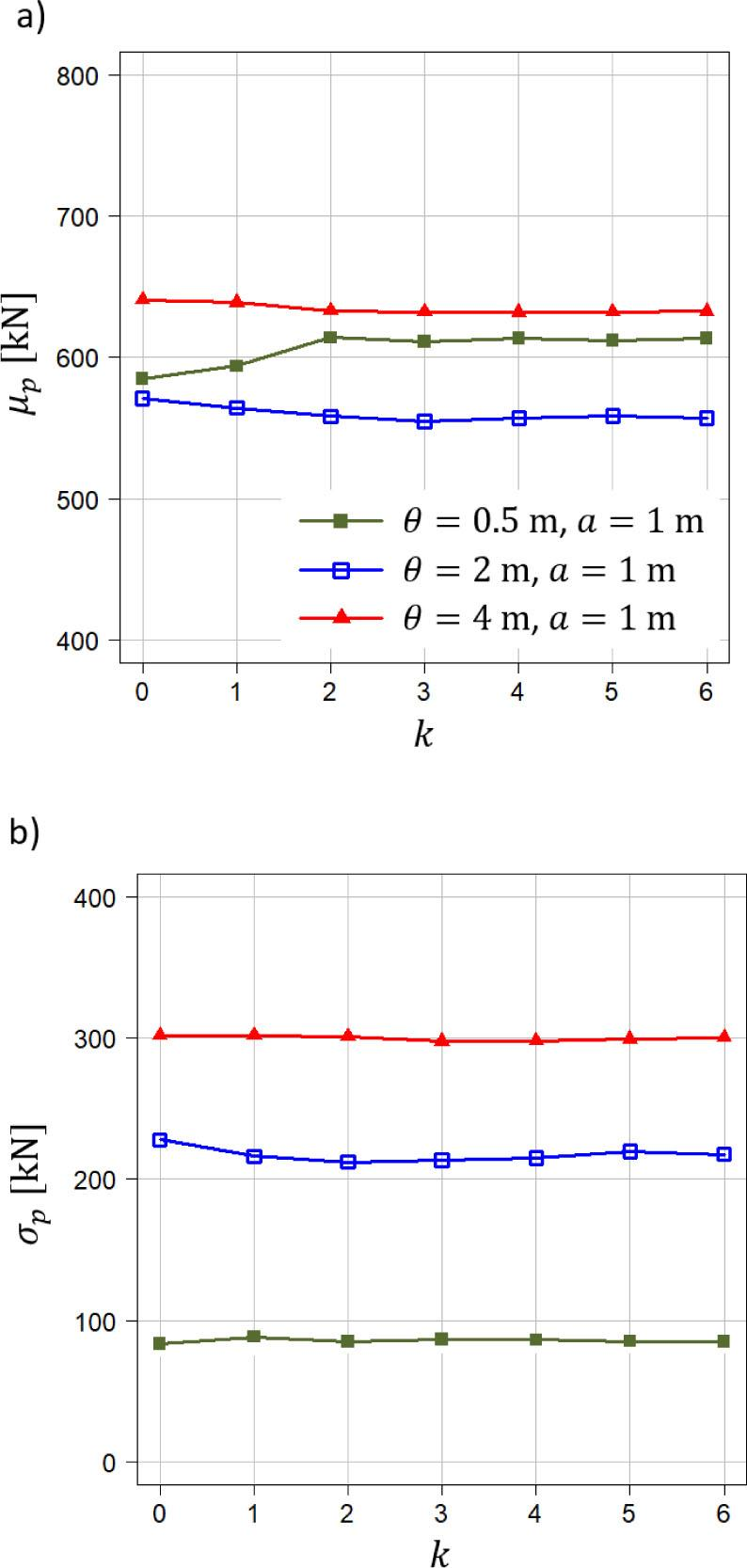Bearing capacity mean values as a function of covariance matrix iteration number (a). Bearing capacity standard deviations as a function of covariance matrix iteration number (b). Case k=0 is for the constant covariance matrix. Results for a square foundation of size 1 m × 1 m.

As shown in Fig. 7, the bearing capacity mean values are in a narrow range, the greatest differences occur in a range of k from 0 to 2; however, the bearing capacity mean values are almost constant for k from 2 to 6. This means that further covariance matrix iteration has no impact on the mean value estimates. The analogous situation is observed in the case of bearing capacity standard deviation, as shown in Fig. 7b. Analogous results for rectangular footing are shown in Fig. 8. In Fig. 8a and Fig, 8b, the bearing capacity mean value and bearing capacity standard deviations are shown, respectively.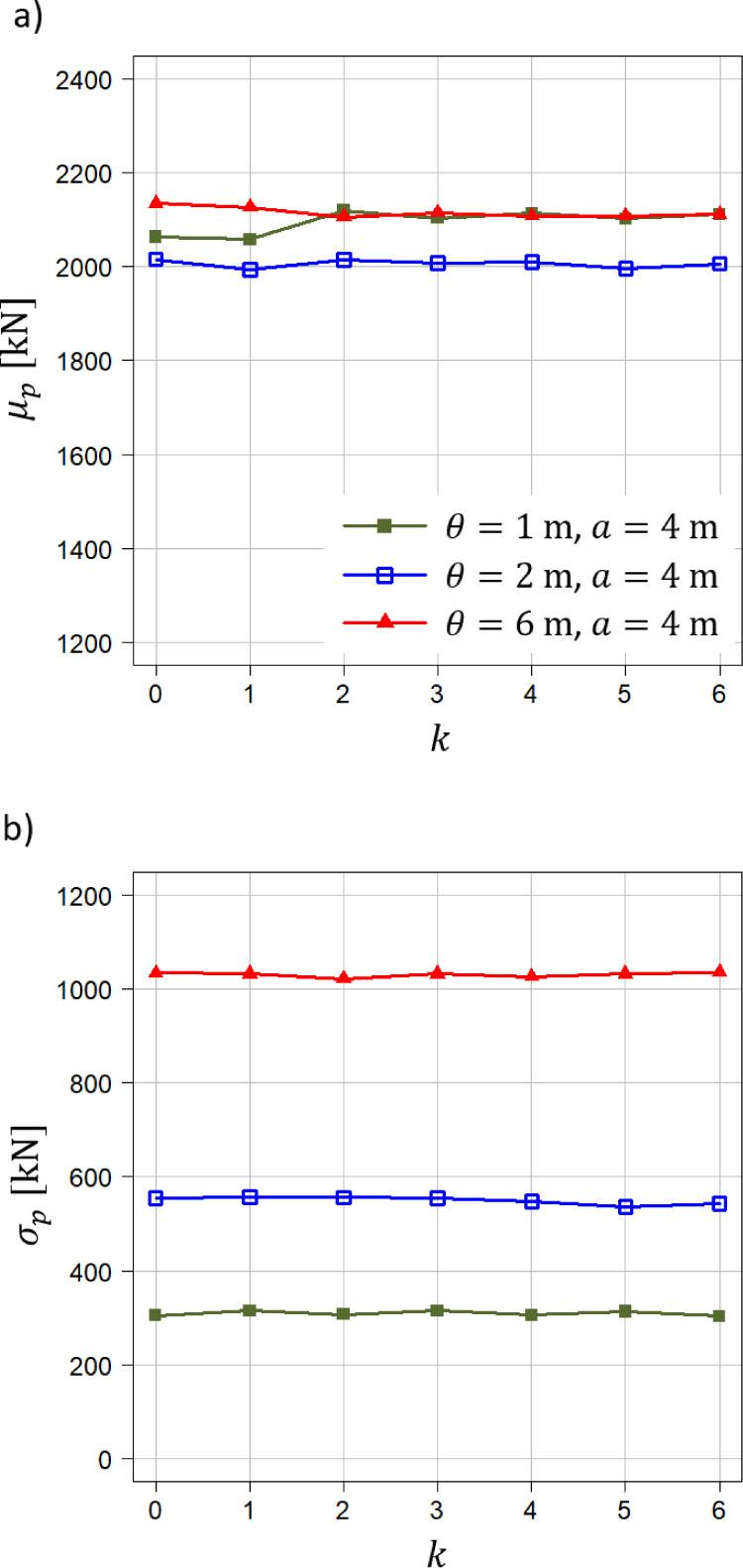Bearing capacity mean values as a function of covariance matrix iteration number (a). Bearing capacity standard deviation as a function of covariance matrix iteration number (b). Case k=0 is for the constant covariance matrix. Results for a rectangular foundation of size 1 m × 4 m.

As shown in Fig. 8, both the bearing capacity mean value and standard deviation are very close to each other for all considered values of k. The greatest differences are observed for the bearing capacity mean values for the scale of fluctuation θ=1 m and θ=0.5 m for the case k=0, and k≥2; and case k=1, and k≥2.

Discussion on the results

The results obtained in the two previous sections are for different applications of the random failure mechanism method (RFMM). The first concerns the bearing capacity of two-layered soil when the failure mechanism is based on two-dimensional simplification, and the second concerns the bearing capacity of the rectangular foundation in the fully three-dimensional case. In both cases, soil spatial variability is considered three-dimensional. According to the obtained results, the differences between the approach with constant covariance matrix ‘C’ (k=0) and the iterative approach ‘A’ (k=1, k=2, k=3, k=4, k=5, and k=6) are generally in a narrow range. As expected, the greatest differences are observed between the constant covariance matrix approach and the iterative one for the first two iterations of the covariance matrix. However, despite the stabilisation of bearing capacity mean values and standard deviation for two and more iterations (k), some fluctuations can be observed, as shown in Fig. 4, Fig. 5, Fig. 7, and Fig. 8. The range of this fluctuation is not important for this study and does not impact the conclusions that can be drawn based on the shown examples. In the author's opinion there are two main sources for this fluctuation in the case of iteration indexes greater than 2, the first possibility is the numerical accuracy, especially in the calculation of high-order integrals, which is calculated to obtain the covariance matrix, another issue is the accuracy of the optimization procedure, which finds approximation to the global minimum of the objective function, not the exact global minimum. The second source can be caused by small modifications of the covariance matrix that are introduced to ensure the positive definiteness of the matrix (the modification occurs if the matrix is not positive definite). In the author's opinion, those factors combined may cause the observed small fluctuations in bearing capacity, mean values and standard deviation. The final accuracy can be influenced also, by the way, the described algorithms work. It can be observed especially for the longer foundations for which the averaging region can be significantly greater than the scale of fluctuation. Therefore, one additional assumption that is not discussed earlier has to be introduced here, that the foundation is rigid. Consequently, it is assumed by default in the numerical examples described above that the wall rests on the foundation and provides infinite foundation stiffness. As a result, the soil strength parameters can be determined as averages of large regions. Certainly, all above mentioned uncerntenties may overlap in the iterative procedure. In Fig. 9 a dependency of bearing capacity mean values, standard deviations and variation coefficient on undrained shear strength coefficinat of variation are shown. The case of two-layered soil is considered. To maintain the clarity of the plot only results for constant covariance matrix (k=0) and the last result for iterative procedure (k=6) are shown in Fig. 9. Three values of cu coefficient of variation are analysed; namely, vcu=0.25, vcu=0.5 and vcu=1.0. A very slow increase in the differences between mean values and standard deviations of bearing capacity in both cases (k=0 and k=6) is observed with the increase of vcu (see Fig. 9a and Fig. 9b). Despite those differances, the resulting bearing capacity coefficient of variation is almost the same for each considered vcu (see Fig. 9c).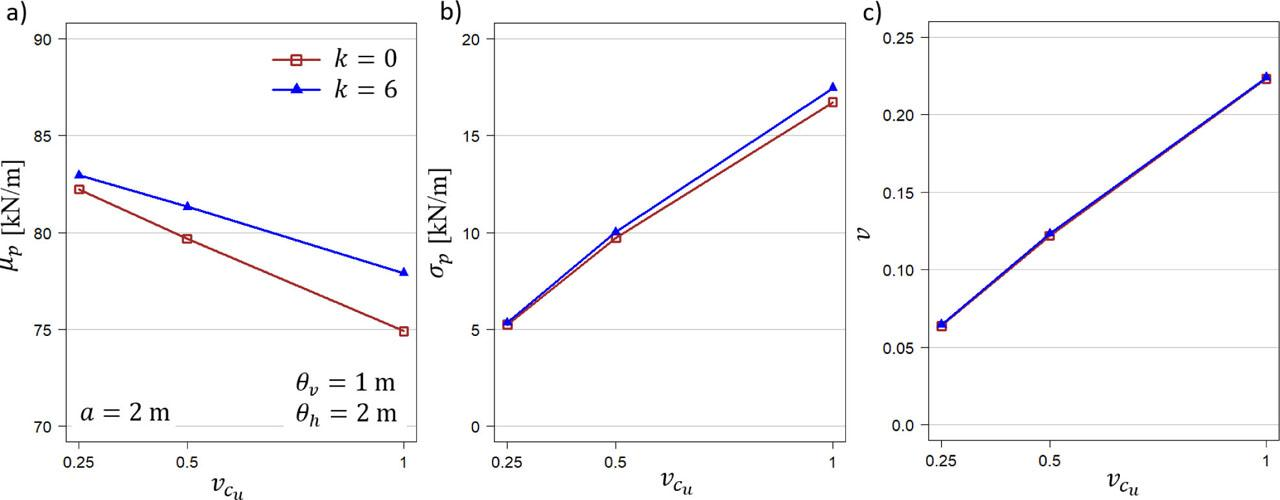Bearing capacity mean values (a), standard deviations (b), and coefficient of variations (c) as a function of coefficient of variation of undrained shear strength. Two-layered soil considered with homogenous top layer (all parameters not mentioned here are the same as for earlier analyses).

All numerical results shown in this study were performed for relatively small number of Monte Carlo realisations. However, as mentioned earlier in this study the main goal is to examine the impact of using more iterations of the covariance matrix. For this particular approach the accurate determination of bearing capacity mean value and standard deviations is not necessary. Despite this, in Fig. 10 an example of such analyses is shown to demonstrate that even relatively low number of Monte Carlo realisations (N = 200) provides quite good estimation of bearing capacity mean values and standard deviations. The conclusions from the study are presented in the next section.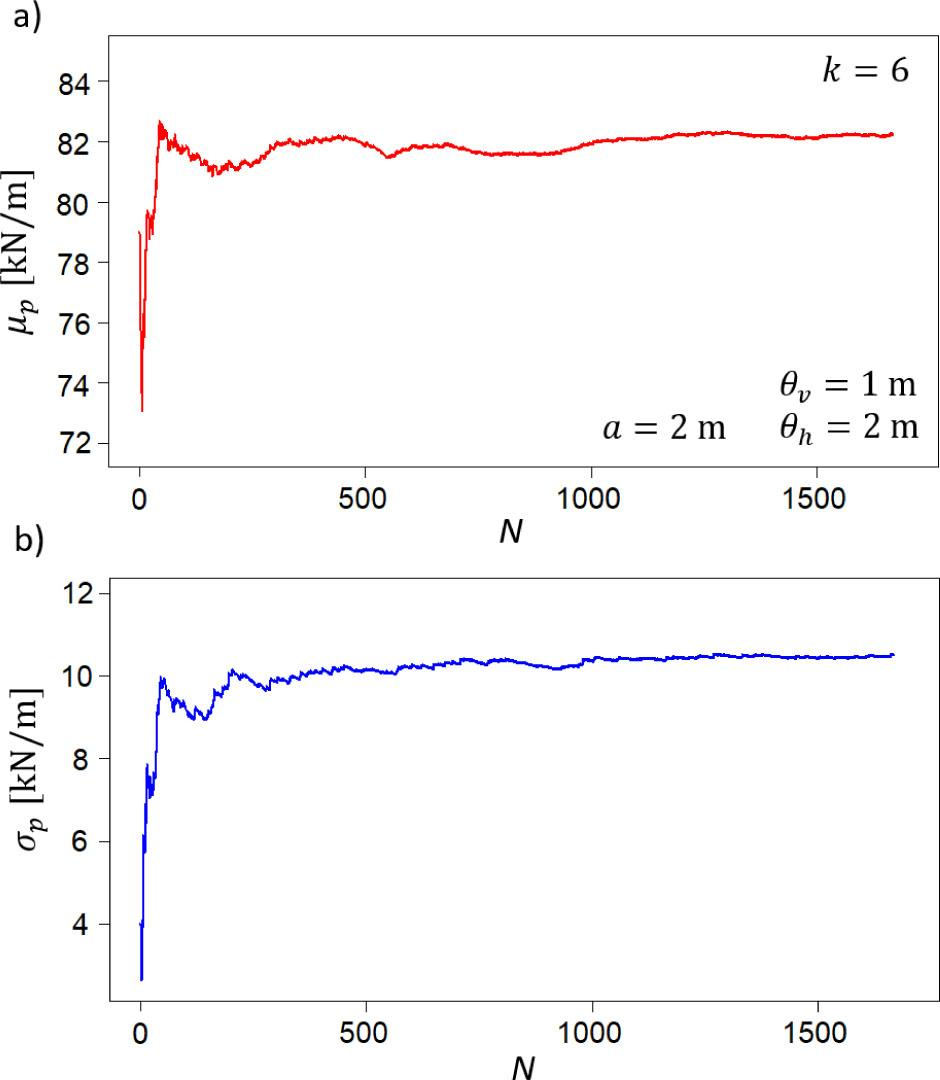Stabilisation of bearing capacity mean values (a) and standard deviations (b) for the iterative approache for k=6.
Conclusions and final remarks

The study propose an original iterative algorithm (path ‘A’ or ‘B’ in Fig. 2) for the upper bound analyses of geotechnical problems with consideration of soil strength spatial variability. The resulting limit loads are realisations of a random variable for which probabilistic characteristics must be determined using the Monte Carlo method. The use of the iterative algorithm ensures the consistency between the failure geometry and the covariance matrix, from which the average soil strength parameters are determined - the averaging procedure is following Vanmarcke's spatial averaging (Vanmarcke, 1977a, 1977b, 1983). Moreover, the general algorithm for using a constant covariance matrix is presented in the study. Performing an analysis via the algorithm based on the constant covariance matrix (see algorithm ‘C’ in Fig. 2) provides a dramatic improvement in computational efficiency. Therefore, the possibility of using a constant covariance matrix is very promising for practical applications and three-dimensional analyses. The main objective of the study is to investigate weather these algorithms give similar results. According to the delivered examples it is shown that, the constant covariance matrix approach provides satisfactory results. However, the investigation required a comparison of the results provided by both approaches. This was done through the iterative algorithm proposed in this study. However, despite good agreement between the two considered approaches for the analysesd scenarios, i.e., bearing capacity of rectangular foundation and two-layered soil under plane strain assumption, other geotechnical applications need to be verified individually. Therefore, the conclusions are only limited to those two scenarios.

The proposed iterative algorithm was utilised in this study to evaluate the bearing capacity of shallow foundations. Two cases were considered, i.e., the bearing capacity of two-layered soil, and the three-dimensional analyses, both were performed for a variety of foundation shapes and scales of fluctuation. To summarize, the following conclusions can be drawn:

The study presents an original iterative algorithm for upper bound analyses of geotechnical problems with the inclusion of soil strength spatial variability. The proposed algorithm can be applied not only to bearing capacity evaluation, as shown in the study, but also for other applications like slope stability or retaining wall analyses. The use of the iterative procedure ensures consistency between the failure geometry and the covariance matrix; therefore, the algorithm allows the recognition and control of the impact of this issue on the resulting estimates. Basing the iterative algorithm on the upper bound theorem provides the opportunity to utilise it as a reference for other probabilistic methods.

This paper shows the possibility of applying the iterative algorithm for shallow foundation random bearing capacity evaluation. Moreover, the proposed algorithm allows the authentication of previous results (e.g., Chwała, 2019; Chwała and Puła, 2020) and indicates that for the undrained conditions, two iterations (k=3 in the algorithm ‘A’ shown in Fig. 2) for the covariance matrix determination is sufficient to obtain stabilisation of the results. Additionally, the influence of the iteration number is limited, and thus, the results obtained for a small number of iterations and a constant covariance matrix are trustworthy.

Scenarios analysed by the iterative algorithm were analysed again using the algorithm based on the constant covariance matrix (see the algorithm ‘C’ in Fig. 2). The obtained results are presented to allow easy comparison between both approaches (see Figs 4, 5, and Fig. 7, 8). The results provided by the algorithm, which use the constant covariance matrix concept, are very close to those obtained by the iterative procedure. This is very promising for further application of the algorithm ‘C’ in solving practical problems, e.g., optimal placement of soil soundings (Chwała, 2020; Chwała, 2021). It is also the most important result of the study that opens up the possibility of using the constant covariance matrix. Thus, a dramatic improvement in the computational efficiency is possible (analysing one realisation of a three-dimensional bearing capacity problem including soil strength spatial variability, takes about 0.5 s for a standard notebook). To summarize, the algorithm based on the constant covariance matrix can be used for three-dimensional random bearing capacity problems and other issues that require high computational efficiency.

Au SK, Beck L. Estimation of small failure probabilities in high dimensions by subset simulation. Probabilistic Engineering Mechanics; 2001; 16:263–277. AuSK BeckL Estimation of small failure probabilities in high dimensions by subset simulation Probabilistic Engineering Mechanics 2001 16 263 277 10.1016/S0266-8920(01)00019-4 Search in Google Scholar

Bagińska I, Kawa M, Janecki W. Estimation of spatial variability of lignite mine dumping ground soil properties using CPTu results. Studia Geotechnica et Mechanica; 2016; 38(1), 3–13. BagińskaI KawaM JaneckiW Estimation of spatial variability of lignite mine dumping ground soil properties using CPTu results Studia Geotechnica et Mechanica 2016 38 1 3 13 10.1515/sgem-2016-0001 Search in Google Scholar

Bagińska I, Kawa M, Łydżba D, Identification of soil types and their arrangement in overburden heaps using the deconvolution approach and CPTu test results. Engineering Geology 276, 105759 BagińskaI KawaM ŁydżbaD Identification of soil types and their arrangement in overburden heaps using the deconvolution approach and CPTu test results Engineering Geology 276 105759 10.1016/j.enggeo.2020.105759 Search in Google Scholar

Ching J, Wu TJ, Stuedlein AW, Bong T. Estimating horizontal scale of fluctuation with limited CPT soundings. Geoscience Frontiers; 2018; Vol. 9, 6, 1597–1608. https://doi.org/10.1016/j.gsf.2017.11.008 ChingJ WuTJ StuedleinAW BongT Estimating horizontal scale of fluctuation with limited CPT soundings Geoscience Frontiers 2018 9 6 1597 1608 https://doi.org/10.1016/j.gsf.2017.11.008 10.1016/j.gsf.2017.11.008 Search in Google Scholar

Chwała M. (2019). Undrained bearing capacity of spatially random soil for rectangular footings. Soils and Foundations, Volume 59, Issue 5, 1508–1521. https://doi.org/10.1016/j.sandf.2019.07.005 ChwałaM. 2019 Undrained bearing capacity of spatially random soil for rectangular footings Soils and Foundations 59 5 1508 1521 https://doi.org/10.1016/j.sandf.2019.07.005 10.1016/j.sandf.2019.07.005 Search in Google Scholar

Chwała M, Puła W (2020). Evaluation of shallow foundation bearing capacity in the case of a two-layered soil and spatial variability in soil strength parameters. PLoS ONE 15(4): e0231992. https://doi.org/10.1371/journal.pone.0231992 ChwałaM PułaW 2020 Evaluation of shallow foundation bearing capacity in the case of a two-layered soil and spatial variability in soil strength parameters PLoS ONE 15 4 e0231992 https://doi.org/10.1371/journal.pone.0231992 10.1371/journal.pone.0231992719014832348332 Search in Google Scholar

Chwała M., (2020). On determining the undrained bearing capacity coefficients of variation for foundations embedded on spatially variable soil. Studia Geotechnica et Mechanica, 2020, 42(2); 125–136. 10.2478/sgem-2019-0037 ChwałaM. 2020 On determining the undrained bearing capacity coefficients of variation for foundations embedded on spatially variable soil Studia Geotechnica et Mechanica 2020 42 2 125 136 10.2478/sgem-2019-0037

Chwała M., (2020). Soil sounding location optimisation for spatially variable soil. Geotechnique Letters 10, 1–10. https://doi.org/10.1680/jgele.20.00012 ChwałaM. 2020 Soil sounding location optimisation for spatially variable soil Geotechnique Letters 10 1 10 https://doi.org/10.1680/jgele.20.00012 10.1680/jgele.20.00012 Search in Google Scholar

Chwała M., (2021). Optimal placement of two soil soundings for rectangular footings. Journal of Rock Mechanics and Geotechnical Engineering, Volume 13, Issue 3, 603–611 https://doi.org/10.1016/j.jrmge.2021.01.007 ChwałaM. 2021 Optimal placement of two soil soundings for rectangular footings Journal of Rock Mechanics and Geotechnical Engineering 13 3 603 611 https://doi.org/10.1016/j.jrmge.2021.01.007 10.1016/j.jrmge.2021.01.007 Search in Google Scholar

Chwała M, Kawa M, (2021). Random failure mechanism method for working platform bearing capacity assessment with a linear trend in undrained shear strength. Journal of Rock Mechanics and Geotechnical Engineering. https://doi.org/10.1016/j.jrmge.2021.06.004 ChwałaM KawaM 2021 Random failure mechanism method for working platform bearing capacity assessment with a linear trend in undrained shear strength Journal of Rock Mechanics and Geotechnical Engineering https://doi.org/10.1016/j.jrmge.2021.06.004 10.1016/j.jrmge.2021.06.004 Search in Google Scholar

Fenton GA, Griffiths DV, (2003). Bearing-capacity prediction of spatially random c ϕ soils. Canadian geotechnical journal, 40(1), 54–65. https://doi.org/10.1139/t02-086 FentonGA GriffithsDV 2003 Bearing-capacity prediction of spatially random c ϕ soils Canadian geotechnical journal 40 1 54 65 https://doi.org/10.1139/t02-086 10.1139/t02-086 Search in Google Scholar

Fenton GA, Griffiths DV. Risk assessment in geotechnical engineering. Wiley; 2008. FentonGA GriffithsDV Risk assessment in geotechnical engineering Wiley 2008 10.1002/9780470284704 Search in Google Scholar

Huang J, Lyamin AV, Griffiths DV, Sloan SW, Krabbenhoft K, Fenton GA. (2013). Undrained bearing capacity of spatially random clays by finite elements and limit analysis. Proceedings of the 18th ICSMGE; Paris 2013;731–734. HuangJ LyaminAV GriffithsDV SloanSW KrabbenhoftK FentonGA 2013 Undrained bearing capacity of spatially random clays by finite elements and limit analysis Proceedings of the 18th ICSMGE Paris 2013 731 734 Search in Google Scholar

Ferreira V, Panagopulos T, Andrade R, Guerrero C, Loures L. (2015). Spatial variability of soil properties and soil erodibility in the Alqueva reservoir watershed. Soild Earth, 6, 383–392. FerreiraV PanagopulosT AndradeR GuerreroC LouresL 2015 Spatial variability of soil properties and soil erodibility in the Alqueva reservoir watershed Soild Earth 6 383 392 10.5194/se-6-383-2015 Search in Google Scholar

Ghanem R, Brzakała W, (1996). Stochastic Finite-Element Analysis of Soil Layers with Random Interface. Journal of Engineering Mechanics, Vol. 122, Issue 4 (April 1996), https://doi.org/10.1061/(ASCE)0733-9399(1996)122:4(361) GhanemR BrzakałaW 1996 Stochastic Finite-Element Analysis of Soil Layers with Random Interface Journal of Engineering Mechanics 122 4 April 1996 https://doi.org/10.1061/(ASCE)0733-9399(1996)122:4(361) 10.1061/(ASCE)0733-9399(1996)122:4(361) Search in Google Scholar

Griffiths DV, Fenton GA, Manoharan N, (2002). Bearing Capacity of Rough Rigid Strip Footing on Cohesive Soil: Probabilistic Study. Journal of Geotechnical and Geoenvironmental Engineering, 2002, 128(9): 743–755. https://doi.org/10.1061/(ASCE)1090-0241(2002)128:9(743) GriffithsDV FentonGA ManoharanN 2002 Bearing Capacity of Rough Rigid Strip Footing on Cohesive Soil: Probabilistic Study Journal of Geotechnical and Geoenvironmental Engineering 2002 128 9 743 755 https://doi.org/10.1061/(ASCE)1090-0241(2002)128:9(743) 10.1061/(ASCE)1090-0241(2002)128:9(743) Search in Google Scholar

Griffiths DV, Fenton GA, (2004). Probabilistic slope stability analysis by finite elements Journal of Geotechnical and Geoenvironmental Engineering, 130 (5) (2004), pp. 507–518, 10.1061/(ASCE)1090-0241(2004)130:5(507) GriffithsDV FentonGA 2004 Probabilistic slope stability analysis by finite elements Journal of Geotechnical and Geoenvironmental Engineering 130 5 2004 507 518 10.1061/(ASCE)1090-0241(2004)130:5(507)

Halder K, Chakraborty D, (2019). Probabilistic bearing capacity of strip footing on reinforced soil slope. Computers and Geotechnics, 2019, 116: 103213. https://doi.org/10.1016/j.compgeo.2019.103213 HalderK ChakrabortyD 2019 Probabilistic bearing capacity of strip footing on reinforced soil slope Computers and Geotechnics 2019 116 103213. https://doi.org/10.1016/j.compgeo.2019.103213 10.1016/j.compgeo.2019.103213 Search in Google Scholar

Halder K, Chakraborty D, (2020). Influence of soil spatial variability on the response of strip footing on geocell-reinforced slope. Computers and Geotechnics, Volume 122, 2020, 103533, https://doi.org/10.1016/j.compgeo.2020.103533. HalderK ChakrabortyD 2020 Influence of soil spatial variability on the response of strip footing on geocell-reinforced slope Computers and Geotechnics 122 2020 103533, https://doi.org/10.1016/j.compgeo.2020.103533. 10.1016/j.compgeo.2020.103533 Search in Google Scholar

Horn RA, Johnson CR. Matrix Analysis. Cambridge University Press 1985. HornRA JohnsonCR Matrix Analysis Cambridge University Press 1985 10.1017/CBO9780511810817 Search in Google Scholar

Juan C. Viviescas, Álvaro J. Mattos & Juan P. Osorio (2020) Uncertainty quantification in the bearing capacity estimation for shallow foundations in sandy soils, Georisk: Assessment and Management of Risk for Engineered Systems and Geohazards, DOI: 10.1080/17499518.2020.1753782 ViviescasJuan C. MattosÁlvaro J. OsorioJuan P. 2020 Uncertainty quantification in the bearing capacity estimation for shallow foundations in sandy soils Georisk: Assessment and Management of Risk for Engineered Systems and Geohazards 10.1080/17499518.2020.1753782

Kasama K, Whittle AJ, (2011). Bearing Capacity of Spatially Random Cohesive Soil Using Numerical Limit Analyses. Journal of Geotechnical and Geoenvironmental Engineering, 2011, 137(11): 989–996. https://doi.org/10.1061/(ASCE)GT.1943-5606.0000531 KasamaK WhittleAJ 2011 Bearing Capacity of Spatially Random Cohesive Soil Using Numerical Limit Analyses Journal of Geotechnical and Geoenvironmental Engineering 2011 137 11 989 996 https://doi.org/10.1061/(ASCE)GT.1943-5606.0000531 10.1061/(ASCE)GT.1943-5606.0000531 Search in Google Scholar

Kawa M, Bagińska I, Wyjadłowski M. Reliability analysis of sheet pile wall in spatially variable soil including CPTu test results. Archives of Civil and Mechanical Engineering; 2019; 19(2):598–613. KawaM BagińskaI WyjadłowskiM Reliability analysis of sheet pile wall in spatially variable soil including CPTu test results Archives of Civil and Mechanical Engineering 2019 19 2 598 613 10.1016/j.acme.2018.10.007 Search in Google Scholar

Kawa M, Puła W. (2020). 3D bearing capacity probabilistic analyses of footings on spatially variable c–ϕ soil. Acta Geotechnica (2020) 15:1453–1466. https://doi.org/10.1007/s11440-019-00853-3 KawaM PułaW 2020 3D bearing capacity probabilistic analyses of footings on spatially variable c–ϕ soil Acta Geotechnica 2020 15 1453 1466 https://doi.org/10.1007/s11440-019-00853-3 10.1007/s11440-019-00853-3 Search in Google Scholar

Kirkpatrick S, Gelatt CD, Vecchi MP. Optimization by Simulated Annealing. Science; 1983; 220, 671–680. KirkpatrickS GelattCD VecchiMP Optimization by Simulated Annealing Science 1983 220 671 680 10.1126/science.220.4598.671 Search in Google Scholar

Kirkpatrick S. Optimization by Simulated Annealing: Quantitative Studies. Journal of Statistical Physics; 1984; Vol. 34, Nos. 5/6. KirkpatrickS Optimization by Simulated Annealing: Quantitative Studies Journal of Statistical Physics 1984 34 5/6 10.1007/BF01009452 Search in Google Scholar

Li Y, Fenton GA, Hicks MA, Xu N, (2021). Probabilistic Bearing Capacity Prediction of Square Footings on 3D Spatially Varying Cohesive Soils. Journal of Geotechnical and Geoenvironmental Engineering 147 (6), 04021035 LiY FentonGA HicksMA XuN 2021 Probabilistic Bearing Capacity Prediction of Square Footings on 3D Spatially Varying Cohesive Soils Journal of Geotechnical and Geoenvironmental Engineering 147 6 04021035 10.1061/(ASCE)GT.1943-5606.0002538 Search in Google Scholar

Li J, Wu C, Luo W, Sun L, White DJ, (2021). An extended Prandtl solution for analytical modelling of the bearing capacity of a shallow foundation on a spatially variable undrained clay. Géotechnique, https://doi.org/10.1680/jgeot.20.P.118 LiJ WuC LuoW SunL WhiteDJ 2021 An extended Prandtl solution for analytical modelling of the bearing capacity of a shallow foundation on a spatially variable undrained clay Géotechnique https://doi.org/10.1680/jgeot.20.P.118 10.1680/jgeot.20.P.118 Search in Google Scholar

Phoon KK, Kulhawy FH, (1999). Characterization of geotechnical variability. Canadian Geotechnical Journal, 36(4), 612–624. https://doi.org/10.1139/t99-038 PhoonKK KulhawyFH 1999 Characterization of geotechnical variability Canadian Geotechnical Journal 36 4 612 624 https://doi.org/10.1139/t99-038 10.1139/t99-038 Search in Google Scholar

Pieczyńska-Kozłowska JM, Puła W, Vessia G. A collection of fluctuation scale values and autocorrelation functions of fine deposits in Emilia Romagna plain (Italy) Geo-Risk 2017 in ASCE Geotechnical Special Publication, 284 (2017), pp. 290–299 Pieczyńska-KozłowskaJM PułaW VessiaG A collection of fluctuation scale values and autocorrelation functions of fine deposits in Emilia Romagna plain (Italy) Geo-Risk 2017 in ASCE Geotechnical Special Publication 284 2017 290 299 Search in Google Scholar

Pieczyńska-Kozłowska JM, Puła W, Chwała M. Search for the worst-case correlation length in the bearing capacity probability of failure analyses. Geo-Risk 2017 in ASCE Geotechnical Special Publication, GSP 283, 534–544. Pieczyńska-KozłowskaJM PułaW ChwałaM Search for the worst-case correlation length in the bearing capacity probability of failure analyses Geo-Risk 2017 in ASCE Geotechnical Special Publication, GSP 283 534 544 Search in Google Scholar

Pramanik, R., Baidya, D.K. & Dhang, N, (2020). Reliability analysis for bearing capacity of surface strip footing using fuzzy finite element method. Geomechanics and Geoengineering: An International Journal, 2020, 15(1): 29–41. https://doi.org/10.1080/17486025.2019.1601268 PramanikR. BaidyaD.K. DhangN 2020 Reliability analysis for bearing capacity of surface strip footing using fuzzy finite element method Geomechanics and Geoengineering: An International Journal 2020 15 1 29 41 https://doi.org/10.1080/17486025.2019.1601268 10.1080/17486025.2019.1601268 Search in Google Scholar

Pramanik, R., Baidya, D.K. & Dhang, N, (2021). Reliability assessment of three-dimensional bearing capacity of shallow foundation using fuzzy set theory. Front. Struct. Civ. Eng. (2021). https://doi.org/10.1007/s11709-021-0698-8 PramanikR. BaidyaD.K. DhangN 2021 Reliability assessment of three-dimensional bearing capacity of shallow foundation using fuzzy set theory Front. Struct. Civ. Eng. 2021 https://doi.org/10.1007/s11709-021-0698-8 10.1007/s11709-021-0698-8 Search in Google Scholar

Puła W. Applications of structural reliability theory to foundations safety evaluation. Wrocław 2004; Wroclaw University of Technology Press [in Polish]. PułaW Applications of structural reliability theory to foundations safety evaluation Wrocław 2004 Wroclaw University of Technology Press [in Polish]. Search in Google Scholar

Puła W. On some aspects of reliability computations in bearing capacity of shallow foundations. In: Griffiths DV, Fenton Gordon A, editors. Puła in: probabilistic methods in geotechnical engineering. CISM courses and lectures, Wien, New York: Springer; 2007; No. 491, 127–45. PułaW On some aspects of reliability computations in bearing capacity of shallow foundations In: GriffithsDV FentonGordon A editors. Puła in: probabilistic methods in geotechnical engineering CISM courses and lectures, Wien, New York Springer 2007 491 127 45 10.1007/978-3-211-73366-0_5 Search in Google Scholar

Puła W, Chwała M, On spatial averaging along random slip lines in the reliability computations of shallow strip foundations. Computers and Geotechnics; 2015; 68, 128–136. PułaW ChwałaM On spatial averaging along random slip lines in the reliability computations of shallow strip foundations Computers and Geotechnics 2015 68 128 136 10.1016/j.compgeo.2015.04.001 Search in Google Scholar

Puła W, Chwała M. Random bearing capacity evaluation of shallow foundations for asymmetrical failure mechanisms with spatial averaging and inclusion of soil self-weight. Computers and Geotechnics; 2018; 101, 176–195. PułaW ChwałaM Random bearing capacity evaluation of shallow foundations for asymmetrical failure mechanisms with spatial averaging and inclusion of soil self-weight Computers and Geotechnics 2018 101 176 195 10.1016/j.compgeo.2018.05.002 Search in Google Scholar

Rainer J, Szabowicz H, (2020). Analysis of underground stratification based on CPTu profiles using high-pass spatial filter. Studia Geotechnica et Mechanica. 2020, s. 1–11. RainerJ SzabowiczH 2020 Analysis of underground stratification based on CPTu profiles using high-pass spatial filter Studia Geotechnica et Mechanica 2020 1 11 10.2478/sgem-2020-0002 Search in Google Scholar

Simoes JT, Neves LC, Antao AN, Guerra NMC. Probabilistic analysis of bearing capacity of shallow foundations using three-dimensional limit analyses. International Journal of Computational Methods; 2014; Vol. 11, No. 02, 1342008-1-20. SimoesJT NevesLC AntaoAN GuerraNMC Probabilistic analysis of bearing capacity of shallow foundations using three-dimensional limit analyses International Journal of Computational Methods 2014 11 02 1342008-1-20. 10.1142/S0219876213420085 Search in Google Scholar

Shield RT, Drucker DC. The application of limit analysis to punch-indentation problems. Journal of Applied Mechanics; 1953; 20, 453–460. ShieldRT DruckerDC The application of limit analysis to punch-indentation problems Journal of Applied Mechanics 1953 20 453 460 10.1115/1.4010747 Search in Google Scholar

Srivastava AGL, Sivakumar BGL, Haldar S, (2010). Influence of spatial variability of permeability property on steady state seepage flow and slope stability analysis. Engineering Geology, 2010, 110(3–4): 93–101. https://doi.org/10.1016/j.enggeo.2009.11.006 SrivastavaAGL SivakumarBGL HaldarS 2010 Influence of spatial variability of permeability property on steady state seepage flow and slope stability analysis Engineering Geology 2010 110 3–4 93 101 https://doi.org/10.1016/j.enggeo.2009.11.006 10.1016/j.enggeo.2009.11.006 Search in Google Scholar

Stuedlein AW, Kramer SL, Arduino P, Holtz RD, (2012). Geotechnical Characterization and Random Field Modeling of Desiccated Clay. Journal of Geotechnical and Geoenvironmental Engineering, 138(11), 1301–1313. https://doi.org/10.1061/(ASCE)GT.1943-5606.0000723 StuedleinAW KramerSL ArduinoP HoltzRD 2012 Geotechnical Characterization and Random Field Modeling of Desiccated Clay Journal of Geotechnical and Geoenvironmental Engineering 138 11 1301 1313 https://doi.org/10.1061/(ASCE)GT.1943-5606.0000723 10.1061/(ASCE)GT.1943-5606.0000723 Search in Google Scholar

The MathWorks. MATLAB R2017b; 2017; Natick. The MathWorks MATLAB R2017b; 2017 Natick Search in Google Scholar

Vanmarcke E.H. Probabilistic modelling of soil profiles. Journal of the Geotechnical Engineering Division; 1977; Vol. 103, 11, 1227–46. VanmarckeE.H. Probabilistic modelling of soil profiles Journal of the Geotechnical Engineering Division 1977 103 11 1227 46 10.1061/AJGEB6.0000517 Search in Google Scholar

Vanmarcke EH. Reliability of earth slopes. Journal of the Geotechnical Engineering Division; 1977; Vol. 103, 11, 1247–65. VanmarckeEH Reliability of earth slopes Journal of the Geotechnical Engineering Division 1977 103 11 1247 65 10.1061/AJGEB6.0000518 Search in Google Scholar

Vanmarcke E.H. Random fields – analysis and synthesis. Cambridge 1983: MIT Press. VanmarckeE.H. Random fields – analysis and synthesis Cambridge 1983 MIT Press Search in Google Scholar

Viviescas J.C, Griffiths DV, Osorio JP, (2021). Geological influence on the spatial variability of soils. International Journal of Geotechnical Engineering, 00(00), 1–9. https://doi.org/10.1080/19386362.2021.1888509 ViviescasJ.C GriffithsDV OsorioJP 2021 Geological influence on the spatial variability of soils International Journal of Geotechnical Engineering 00 00 1 9 https://doi.org/10.1080/19386362.2021.1888509 10.1080/19386362.2021.1888509 Search in Google Scholar

Zhu D, Griffiths DV, Huang J, Fenton GA, (2017). Probabilistic stability analyses of undrained slopes with linearly increasing mean strength. Géotechnique, 2017, 67(8): 733–746. https://doi.org/10.1680/jgeot.16.P.223 ZhuD GriffithsDV HuangJ FentonGA 2017 Probabilistic stability analyses of undrained slopes with linearly increasing mean strength Géotechnique 2017 67 8 733 746 https://doi.org/10.1680/jgeot.16.P.223 10.1680/jgeot.16.P.223 Search in Google Scholar

Żyliński K, Korzec A, Winkelmann K, Górski J., (2020). Random Field Model of Foundations at the Example of Continuous Footing. AIP Conf. Proc. 2239, 020052-1–020052-11; https://doi.org/10.1063/5.0007811 ŻylińskiK KorzecA WinkelmannK GórskiJ 2020 Random Field Model of Foundations at the Example of Continuous Footing AIP Conf. Proc. 2239 020052-1 020052-11 https://doi.org/10.1063/5.0007811 10.1063/5.0007811 Search in Google Scholar

• #### Case studies on Q-slope method use for slope stability analyses

Empfohlene Artikel von Trend MD